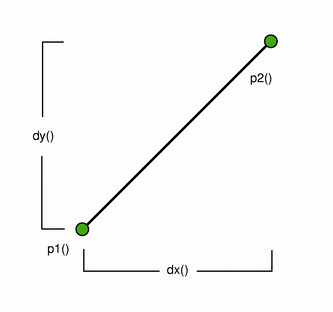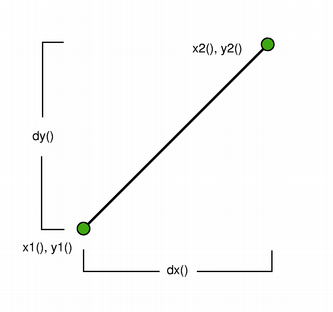# QLine Class

The QLine class provides a two-dimensional vector using integer precision. More...

 Header: #include CMake: find_package(Qt6 REQUIRED COMPONENTS Core) target_link_libraries(mytarget PRIVATE Qt6::Core) qmake: QT += core

## Public Functions

 QLine() QLine(const QPoint &p1, const QPoint &p2) QLine(int x1, int y1, int x2, int y2) QPoint p1() const QPoint p2() const int x1() const int x2() const int y1() const int y2() const QPoint center() const int dx() const int dy() const bool isNull() const void setP1(const QPoint &p1) void setP2(const QPoint &p2) void setLine(int x1, int y1, int x2, int y2) void setPoints(const QPoint &p1, const QPoint &p2) QLineF toLineF() const void translate(const QPoint &offset) void translate(int dx, int dy) QLine translated(const QPoint &offset) const QLine translated(int dx, int dy) const bool operator!=(const QLine &line) const bool operator==(const QLine &line) const
 QDataStream & operator<<(QDataStream &stream, const QLine &line) QDataStream & operator>>(QDataStream &stream, QLine &line)

## Detailed Description

A QLine describes a finite length line (or a line segment) on a two-dimensional surface. The start and end points of the line are specified using integer point accuracy for coordinates. Use the QLineF constructor to retrieve a floating point copy.The positions of the line's start and end points can be retrieved using the p1(), x1(), y1(), p2(), x2(), and y2() functions. The dx() and dy() functions return the horizontal and vertical components of the line. Use isNull() to determine whether the QLine represents a valid line or a null line.

Finally, the line can be translated a given offset using the translate() function.

## Member Function Documentation

### `[constexpr] `QLine::QLine()

Constructs a null line.

### `[constexpr] `QLine::QLine(const QPoint &p1, const QPoint &p2)

Constructs a line object that represents the line between p1 and p2.

### `[constexpr] `QLine::QLine(intx1, inty1, intx2, inty2)

Constructs a line object that represents the line between (x1, y1) and (x2, y2).

### `[constexpr] `QPoint QLine::p1() const

Returns the line's start point.

### `[constexpr] `QPoint QLine::p2() const

Returns the line's end point.

### `[constexpr] `int QLine::x1() const

Returns the x-coordinate of the line's start point.

### `[constexpr] `int QLine::x2() const

Returns the x-coordinate of the line's end point.

### `[constexpr] `int QLine::y1() const

Returns the y-coordinate of the line's start point.

### `[constexpr] `int QLine::y2() const

Returns the y-coordinate of the line's end point.

### `[constexpr] `QPoint QLine::center() const

Returns the center point of this line. This is equivalent to (p1() + p2()) / 2, except it will never overflow.

### `[constexpr] `int QLine::dx() const

Returns the horizontal component of the line's vector.

### `[constexpr] `int QLine::dy() const

Returns the vertical component of the line's vector.

### `[constexpr] `bool QLine::isNull() const

Returns `true` if the line does not have distinct start and end points; otherwise returns `false`.

### void QLine::setP1(const QPoint &p1)

Sets the starting point of this line to p1.

### void QLine::setP2(const QPoint &p2)

Sets the end point of this line to p2.

### void QLine::setLine(intx1, inty1, intx2, inty2)

Sets this line to the start in x1, y1 and end in x2, y2.

### void QLine::setPoints(const QPoint &p1, const QPoint &p2)

Sets the start point of this line to p1 and the end point of this line to p2.

### `[constexpr, since 6.4] `QLineF QLine::toLineF() const

Returns this line as a line with floating point accuracy.

This function was introduced in Qt 6.4.

### void QLine::translate(const QPoint &offset)

Translates this line by the given offset.

### void QLine::translate(intdx, intdy)

Translates this line the distance specified by dx and dy.

### `[constexpr] `QLine QLine::translated(const QPoint &offset) const

Returns this line translated by the given offset.

### `[constexpr] `QLine QLine::translated(intdx, intdy) const

Returns this line translated the distance specified by dx and dy.

### `[constexpr] `bool QLine::operator!=(const QLine &line) const

Returns `true` if the given line is not the same as this line.

A line is different from another line if any of their start or end points differ, or the internal order of the points is different.

### `[constexpr] `bool QLine::operator==(const QLine &line) const

Returns `true` if the given line is the same as this line.

A line is identical to another line if the start and end points are identical, and the internal order of the points is the same.

## Related Non-Members

### QDataStream &operator<<(QDataStream &stream, const QLine &line)

Writes the given line to the given stream and returns a reference to the stream.